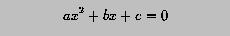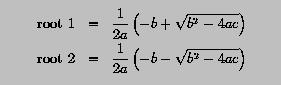# Programming Example: Quadratic Equation Solver### Problem Statement

Given a quadratic equation as follows:if b*b-4*a*c is non-negative, the roots of the equation can be computed with the following formulae:Write a program to read in the coefficients a, b and c, and compute and display the roots. You can assume that b*b - 4*a*c is always non-negative.### Solution

```! ---------------------------------------------------
!   Solve  Ax^2 + Bx + C = 0 given B*B-4*A*C >= 0
! ---------------------------------------------------

IMPLICIT  NONE

REAL  :: a, b, c
REAL  :: d
REAL  :: root1, root2

!  read in the coefficients a, b and c

WRITE(*,*) 'A, B, C Please : '

!  compute the square root of discriminant d

d  = SQRT(b*b - 4.0*a*c)

!  solve the equation

root1 = (-b + d)/(2.0*a)   ! first root
root2 = (-b - d)/(2.0*a)   ! second root

!  display the results

WRITE(*,*)
WRITE(*,*)  'Roots are ', root1, ' and ', root2

```
Click here to download this program.### Program Output

``` A, B, C Please :
1.0 -5.0 3.0

Roots are 4.30277538 and 0.697224379
```

The input to the above problem consists of three real numbers, 1.0, -5.0 and 3.0, and the computed roots are 4.30277538 and 0.697224379.### Discussion

• The WRITE displays a message like this
```A, B, C Please :
```
After displaying this message, the computer executes READ. Since there is no input value, it will wait until the user types in three real values and hits the Return key. Then, these values are stored in a, b and c.
• The first assignment statement computes the square root of the discriminant (i.e., b*b - 4.0*a*c) and stores it into variable d.
• The roots are computed with the second and third assignments. Note that the parenthesis surrounding 2.0*a cannot be removed; otherwise, it is equivalent to ((-b + d)/2.0)*a, which is wrong.
• The last two WRITE statements display the roots.